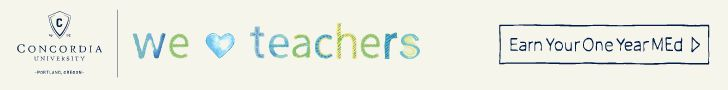# First-Grade Mathematics StandardsIn first grade, students will learn to solve addition and subtraction problems, be able to measure lengths in an indirect way, tell time and understand shapes. Here are a few examples of what your child will study throughout the year, questions to ask his or her teachers and tips for reinforcing math skills at home.

### Operations and Algebraic Thinking

1. Understand the relationship between addition and subtraction. For instance, subtraction is a missing addend problem.
Q: To solve 13 – 6, find the number that makes 13 when added to 6
A: 6 + 7 =13
By knowing that 7 + 5 = 12, you also know that 12 – 5 = 7
2. Subtract and add within 20 and relate it to counting, especially when starting with a number higher than one to count to a second number.
Q: Counting on 3 to add 3
A: 3 + (1 + 1 +1) = 6
3. Making ten (6 + 8 = 6 + 4 + 4 = 10 + 4 = 14) and decomposing numbers leading to ten (14 – 5 = 14 – 4 – 1 = 10 – 1 = 9).
4. Using easier and known sums to create equivalent sums.
5 + 8 = 5 + 5 + 3 = 10 + 3 = 13
5. Understand what the equal sign means and figure out whether addition and subtraction equations are true or false.
Q: Which of these equations is false:
5 = 5
8 = 9 – 1
5 + 3 = 3 + 5
2 + 6 = 5 + 4

A: 2 + 6 = 5 + 4

6. In addition or subtraction equations involving three whole numbers, be able to determine the unknown number.
Q: 2 + 6 + ? = 12
A: 6

Q: 7 = ? – 2
A: 9

Q: 4 + 4 = ?
A: 8

### Number and Operations in Base Ten

1. Be able to count to 120 beginning with any number less than 120. Within this range, represent objects using a written number and read and write numerals.
2. Comprehend that each digit of a two-digit number represents a group of ones and tens.
3. Add numbers within 100, using various strategies based on the relationship between addition and subtraction, place value, or properties of operations.
47 + 8 = 55 (first add the ones place, then add the tens place)
Be able to explain why that strategy was chosen and how it relates to the written problem
4. Comprehend that 2-digit numbers means adding tens and tens and ones and ones, and it is sometimes necessary to compose a ten.
5. Mentally add or subtract 10 from a 2-digit number without counting and be able to explain the strategy used.
86 – 10 = 76 (Only subtract in the tens place)

### Measurements and Data

1. Be able to measure lengths in an indirect way and by paraphrasing lengths of units. For instance, put three objects in order by length (long, longer, longest); indirectly compare lengths of two objects by using a third object (shorter than, longer than)
2. Using both digital and analog clocks, write and tell time by half-hours and hours.3. Organize, represent and interpret data that has up to three categories. Be able to both answer and ask questions about how many total data points there are, how many are in each category and if there are more or less in one category than another.

### Geometry

1. Understand shapes and learn the difference between defining characteristics (squares are four-sided and closed) and nondefining characteristics (size, color, orientation). Draw and build shapes that express defining characteristics.
Q: Draw the figure: six-sided and closed (defining characteristic).
A:2. Create two-dimensional shapes (squares, triangles, rectangles, trapezoids, quarter-circles and half-circles) or three-dimensional shapes (right rectangular prisms, right circular cones, cubes and right circular cylinders) to make a composite shape; create additional new shapes using the composite shape.
3. Parcel rectangles and circles into two or four equal shares and describe the shares using the words quarters, fourths and halves and the phrases quarter of, fourth of and half of. Be able to describe a whole as either two or four of the shares. Understand that decomposing these examples into additional equal shares makes smaller shares.
Q: Split each shape into fourths.
A:Learn More: Click to view related resources.